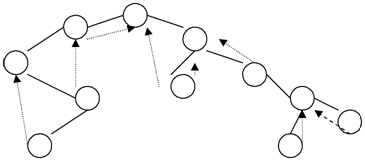## The threaded binary tree, Data Structure & Algorithms

Assignment Help:

By changing the NULL lines in a binary tree to the special links called threads, it is possible to execute traversal, insertion and deletion without using either a stack or recursion.

In a right in threaded binary tree each NULL link is replaced by a particular link to the successor of that node under the inorder traversal called right threaded. Using right threads we shall find it easy to perform an inorder traversal of the tree, since we need to only follow either an ordinary link or a threaded to find the next node to visit.

If we replace each NULL left link by a particular link to the predecessor of the node known as left threaded under inorder traversal the tree is called as left in threaded binary tree. If both the left and right threads are present in tree then it is called as fully threaded binary tree for example:#### Linked List Variations, Part1: Deque and Bag Implementation First, complet...

Part1: Deque and Bag Implementation First, complete the Linked List Implementation of the Deque (as in Worksheet 19) and Bag ADTs (Worksheet 22). Files Needed: linkedList.c Linke

#### Define algorithm, What is an Algorithm? An algorithm is a sequence of u...

What is an Algorithm? An algorithm is a sequence of unambiguous instructions for solving a problem, i.e., for getting a needed output for any legitimate input in a finite amoun

#### Determine the types of java, Determine the types of JAVA Java has two p...

Determine the types of JAVA Java has two parts... 1. Core language -- variables, arrays, objects o Java Virtual Machine (JVM) runs the core language o Core language is

#### Importance of object-oriented over java, Importance of Object-Oriented over...

Importance of Object-Oriented over java Java is basically based on OOP notions of classes and objects. Java uses a formal OOP type system that should be obeyed at compile-t

#### Undirected graph, Graphs are data structures which consist of a set of vert...

Graphs are data structures which consist of a set of vertices & a set of edges which connect the vertices. A graph where the edges are directed is called directed graph. Or else, i

#### What is algorithm, What is Algorithm A finite sequence of steps for a...

What is Algorithm A finite sequence of steps for accomplishing some computational task. An algorithm should Have steps which are simple and definite enough to be done

#### C++ function, Write c++ function to traverse the threaded binary tree in in...

Write c++ function to traverse the threaded binary tree in inorder traversal

#### Implement stack using two queues, How To implement stack using two queues ,...

How To implement stack using two queues , analyze the running time of the stack operations ?

#### General, whats the definition of ADT and data type?

whats the definition of ADT and data type?

#### Rotations in binary tree, H o w can you r ot a t e a B i n a r y...

H o w can you r ot a t e a B i n a r y Tr e e? E x pl a i n r i g h t a n d l eft r ot a tion s by taking an e x a mpl e.   If after